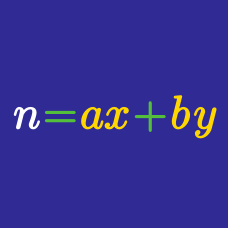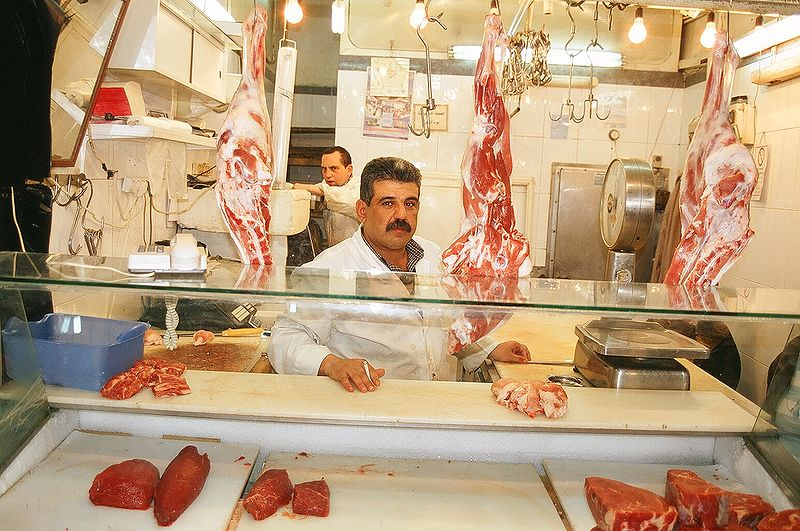Number Theory

# Linear Diophantine Equations: Level 4 Challenges

$\frac { \begin{matrix} & A & B & C & D & E \\ \times & & & & 1 & 2 \end{matrix} }{ C\quad D\quad E\quad 0\quad A\quad B }$

Find $$\overline { ABCDE }$$.

How many possible 6 digit numbers are there of the form $$N=\overline{abcabd}$$ where $$a \neq 0, d \neq 0$$, $$d = c + 1$$ and $$N$$ is a perfect square?

Details and assumptions

The condition of $$a \neq 0$$ follows because we have a 6 digit number.
The condition of $$d \neq 0$$ follows because $$0 \neq 9 + 1$$.At my butcher shop, you can buy 2 pounds of chicken thigh and 1 pound of chicken feet for 738 cents, and 3 pounds of chicken thigh and 1 pound of chicken heart for 852 cents.

For how many ordered sets of integers $$(t, f, h )$$, with each of the element between 0 and 100 inclusive, can you determine the exact total cost of $$t$$ pounds of chicken thigh, $$f$$ pounds of chicken feet, and $$h$$ pounds of chicken heart?

Assume that the cost per pound of any given meat is constant.

McNuggets come in boxes of 7, 13, and 19. What is the largest amount of McNuggets that the customer cannot exactly obtain?

Consider the system of equations

$\begin{split} y & = 2{x}_{1}+{x}_{2} \\ y & = 3{x}_{2}+{x}_{3} \\ y & = 4{x}_{3}+{x}_{4} \\ y & = 5{x}_{4}+{x}_{5} \\ y & = 6{x}_{5}+{x}_{1}. \end{split}$

If all of the variables are integers, what is the minimum positive integer value of

$\left(\sum_{i=1}^{5}{x}_{i}\right) - y ?$

×